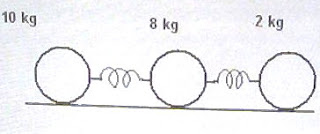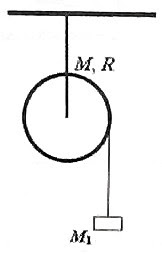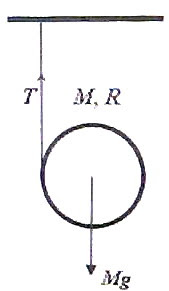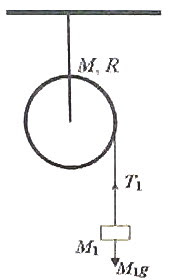## Pages

`“Life is like riding a bicycle.  To keep your balance you must keep moving.”–Albert Einstein`

## Friday, October 30, 2009

### AP Physics B - Free Response Practice Question involving Waves and Electromagnetism

Today I’ll give you a free response practice question involving waves and electromagnetism. Here is the question:

Your teacher wants you to measure the frequency of a sinusoidal alternating current, making use of the resonance condition in a vibrating wire segment. She gives you a sonometer (fig.) with a steel wire of linear density m, standard weights, a small electromagnet for vibrating the wire, an AC source of variable output voltage but of fixed frequency (which is to be measured), connecting wires and metre scale. Now answer the following questions:

(a) Show in a diagram how you will arrange the given items for the measurement of the frequency of the given AC source.

(b) You are given that the frequency of vibration of the sonometer wire segment of length ℓ in the sth mode is (s/2ℓ)√(T/m) where T is the tension.

(i) Now explain briefly how you will proceed to measure the frequency of the alternating current making use of the vibration of the sonometer wire segment in the fundamental mode.

(ii) Write down the expression for the frequency of the wire.

(ii) How is the frequency of AC related to the frequency of vibration of the sonometer wire? Justify your answer.

(c) Your teacher now wants you to use a brass wire of linear density μ instead of the steel wire in the sonometer. Instead of the electromagnet she gives you a sufficiently strong permanent bar magnet.

Show in a diagram how you will arrange the given items for the measurement of the frequency of the given AC source.

(d) (i) Explain briefly how you will proceed to measure the frequency of the alternating current in this case.

(ii) How is the frequency of AC related to the frequency of vibration of the sonometer wire in this case? Justify your answer.

The above question carries 15 points. You have about 17 minutes for answering it. Try to answer the question. Certainly I’ll come back shortly with a model answer for your benefit.

## Tuesday, October 20, 2009

### AP Physics B & C- Multiple Choice Questions (for practice) on Conservation of Energy

Many of life's failures are people who did not realize how close they were to success when they gave up.

– Thomas A.Edison

The following two questions will be beneficial for AP Physics B as well as C:

(1) A rain drop of mass m at a height h starts from rest and gets accelerated under gravity initially. Because of the viscosity of air, its motion is resisted and it finally falls down with a constant terminal velocity vT and hits the ground. You may neglect the force of buoyancy. Assume that the acceleration due to gravity, g is constant and the rain drop moves vertically down over the entire path. The magnitude of the average viscous force on the rain drop during the entire downward trip is

(a) mg

(b) mg – (mvT2/h)

(c) mg + (mvT2/h)

(d) mg + (mvT2/2h)

(e) mg – (mvT2/2h)

The initial gravitational potential energy of the drop at height h is mgh. On reaching the ground, it has lost its gravitational potential energy but has gained kinetic energy equal to ½ mvT2.

The loss of energy because of air resistance is mgh ½ mvT2.

If the magnitude of the average viscous force on the rain drop is F, we have

Fh = mgh ½ mvT2

Therefore, F = mg – (mvT2/2h)

(2) In the above question what is the viscous force when the rain drop moves down with its terminal velocity?

(a) mg upwards

(b) zero

(c) mg downwards

(d) mvT2/h) upwards

(e) mvT2/h) downwards

When the rain drop moves down with its terminal velocity, the net force acting on it is zero. This means that the weight mg of the rain drop and the viscous force offered by air are equal and opposite. Therefore, the viscous force has magnitude mg and is directed upwards.

The following question involves resistive torques in rotational motion.(3) One end of a light inextensible string is tied to a solid cylinder of mass M and radius R. The string is wound round the cylinder many times and a mass m is suspended from the free end of the string so that on releasing the mass, the cylinder rotates about its central axis. There exists an appreciable amount of friction between the cylinder and the axle about which the cylinder rotates. When the mass m which is initially at a height h from the ground (Fig.) is released from rest, it strikes the ground with speed v. How much energy is lost from the system for doing work against friction during the fall of the mass m through the height h?

(a) mgh mv2+ ¼ Mv2)

(b) mgh ½ mv2

(c) mgh ½ (m + M)v2

(d) mgh mv2+ ½ MR2v2)

(e) mgh mv2+ ¼ MR2v2)

The system acquires kinetic energy at the cost of the gravitational potential energy of the suspended mass m.

The initial energy E1 of the system is given by

E1 = mgh.

The final energy E2 of the system at the moment the mass m hits the ground is the sum of the translational kinetic energy of the mass m and the rotational kinetic energy of the cylinder:

E2 = ½ mv2 + ½ (MR2/2)×(v2/R2)

[The second term on the right hand side is ½ 2 where I is the moment of inertia of the cylinder (MR2/2) and ω is its angular velocity (v/R) when the mass m strikes the ground].

Therefore, E2 = ½ mv2 + ¼ Mv2

The work (W) done by the system against frictional forces is given by

W = E1 E2 = mgh – (½ mv2+ ¼ Mv2)

## Thursday, October 8, 2009

### Multiple Choice Practice Questions on Rotation for AP Physics C

Some multiple choice practice questions (with solution) on rotational motion were posted earlier on this site. You can access those posts by clicking on the label ‘rotational motion’ or ‘rotation’ below this post. We will discuss a few more multiple choice questions (MCQ) for practice in this section today:

(1) A car moves forward on level road with uniform velocity of 4 ms–1. If there is no slipping of the tyres, the velocities (with respect to the road) of the points at the bottom, centre and the top of a tyre are respectively

(a) 4 ms–1, 4 ms–1 and 4 ms–1

(b) zero, 4 ms–1and 8 ms–1

(c) 4 ms–1, zero and 8 ms–1

(d) 8 ms–1, 4 ms–1and 8 ms–1

(e) 8 ms–1, 4 ms–1 and zero

The wheel has translational motion as well as rotational motion. Because of the rotation, points at the bottom of the wheel move backward with speed 4 ms–1 where as points at the top move forward with speed 4 ms–1. The resultant speed of points at the bottom is therefore zero (4 ms–1 – 4 ms–1) where as that of points at the top is 8 ms–1 (4 ms–1 + 4 ms–1). The linear velocity of the centre of the wheel because of its rotation is zero and hence the velocity of 4 ms–1 due to the translational motion is preserved at the central point of the wheel. The correct option is (b).(2) Three solid spheres of masses 10 kg, 8 kg and 2 kg are connected by two springs of negligible mass and force constants 10 Nm–1 and 12 Nm–1 as shown. The spheres are at rest on a horizontal frictionless surface so that their centres are along the x-axis. An impulsive force is applied on the 10 kg mass so that it starts moving with a velocity of 2 ms–1 along the positive x-direction. The velocity of the centre of mass of the system of spheres is

(a) 0.5 ms–1

(b) 0.8 ms–1

(c) 1 ms–1

(d) 1.6 ms–1

(e) 2 ms–1

Some of you will be confused by this simple problem. You should remember that the forces developed in the springs are internal forces in the system and hence they will not affect the velocity of the centre of mass. The law of conservation of momentum is obeyed and we can equate the initial momentum to the final momentum:

10×2 + 8×0 + 2×0 = (10+8+2)v where v is the velocity of the centre of mass.

This gives v = 1 ms

(3) A horizontal turn table of mass M in the form of a uniform circular disc of radius R is rotating about a central vertical axis with uniform angular velocity ω. The friction at the axis is negligible. When two equal masses m and m are gently placed symmetrically on either side of the centre at distance R/2 (Fig), the angular velocity of the turn table is reduced to ω/2. Then each mass m is equal to

(a) M/4

(b) M/2

(c) M

(d) 3M/2

(e) 2M

This question is meant for checking your understanding of the law of conservation of angular momentum and for testing whether you can apply it in situations where it is needed.

The angular momentum is conserved in the absence of external torque. The angular momentum of the system before and after placing the two equal masses is the same so that we have

(MR2/2) ω = [(MR2/2) + 2m (R/2)2] ω/2

[Note that MR2/2 is the moment of inertia of the disc about the central axis and 2m (R/2)2 is the total moment of inertia of the two masses m and m about the same axis which is distant R/2 from them].

From the above equation MR2/4 = mR2/4 so that m = M

(4) A solid sphere S1 spinning about its own central axis with angular velocity ω moves along a frictionless horizontal surface and undergoes a head-on elastic collision with an identical solid sphere S2 at rest on the surface. After the collision the spin angular velocities of S1 and S2 will be respectively

(a) ω/2 and ω/2

(b) ω and zero

(c) zero and ω

(d) zero and 2ω

(e) 2ω and zero

Since the line of action of the force exerted by S1 on S2 passes through the centre of S2, there is no torque on S2. Its spin angular momentum after the collision is therefore zero (same as that before the collision). Since the angular momentum of the system is to be conserved, the angular momentum of S1 also is unchanged by the collision. Hence its spin angular velocity after the collision is ω. The correct option is (b).

(5) A car is decelerating uniformly from velocity v1 to velocity v2 while getting displaced by s. If the diameter of the wheel is d, the angular acceleration of the wheel is

(a) (v12 v22)/ds

(b) (v2 v1)/ds

(c) (v1 v2)/ds

(d) (v22 v12)/ds

(e) 2(v22 v12)/ds

The acceleration ‘a of the car is given by

v22 = v12 + 2as

[We have applied the equation of linear motion, v2 = v02 + 2as (or, v2 = u2 + 2as)].

Therefore, a = (v22 v12)/2s.

The angular acceleration ‘α’ of the wheel is given by

α = a/r where r is the radius of the wheel (which is equal to d/2).

Therefore, α = [(v22 v12)/2s]/(d/2) = (v22 v12)/ds

## Sunday, October 4, 2009

### Answer to AP Physics C Free Response Practice Question on Rotation

In the post dated 30th September 2009 A free response question (for practice) on rotational motion for AP Physics C aspirants was given. As promised I give below a model answer for the same. The question also is given:A solid cylinder of mass M and radius R has a light inextensible string wound round it. The free end of the string is tied to a rigid support (Fig.) and the cylinder is released from its state of rest.

(a) Determine the acceleration ‘a of the cylinder as it moves down, unwinding the string.
The cylinder itself is now suspended from the support so that it is free to rotate about its axle. The friction at the axle of the cylinder is negligible. A mass M1 is now attached to the free end of the string wound round the cylinder as shown in the adjoining figure and the system is released from rest at time t = 0. Now answer the following questions (b), (c) and (d):(b) Determine the acceleration a1 of the mass M1 in terms of the given parameters.

(c) In terms of the given parameters obtain the angular acceleration of the cylinder when the mass M1 moves down, unwinding the string.

(d) Determine the kinetic energy of the cylinder at time t.(a) The weight Mg of the cylinder tries to drive it downwards while the tension T in the sring tries to oppose the downward motion (fig.). The net force on the cylinder is MgT. If the downward acceleration is a we have

MgT = Ma from which T = M (ga)

The torque produced by the tension T rotates the cylinder and produces an angular acceleration α (let us say) so that we have

TR = Iα where I is the moment of inertia (MR2/2) of the cylinder about its axis (axle). Therefore, TR = (MR2/2)α

But α = a/R so that TR = (MR2/2)(a/R)

Since T = M (ga), the above equation becomes

M (ga)R = (MR2/2)(a/R) from which (ga) = a/2 so that a = 2g/3(b) The force driving the mass M1 downwards is its weight M1g. The tension T1 in the sring tries to oppose the downward motion (fig.). The downward acceleration a1 of the mass M1 is therefore given by M1gT1 = M1a1 from which

T1 = M1(g – a1)

Since the torque produced by the tension T1 is T1R and this is responsible for the rotation of the cylinder, we have

T1R = Iα1 where α1 is the angular acceleration of the cylinder.

Substituting for T1 and the moment of inertia I, we have

M1(g – a1)R = (MR2/2)α1

Substituting for α1 = a1/R, the above equation becomes

M1(g – a1)R = (MR2/2)(a1/R)

Or, 2M1(g – a1) = Ma1, from which a1 = 2M1g/(2M1+M)

(c) The angular acceleration α1 of the cylinder when the mass M1 moves down, unwinding the string is given by

α1 = a1/R = 2M1g/ [(2M1+M)R]

(d) The kinetic energy of the cylinder at time t is due to its rotational motion and is equal to ½ Iω2 where ω is its angular velocity at time t.

But ω is related to the angular acceleration α1 of the cylinder as

α1 = dω/dt

Therefore, dω/dt = 2M1g/ [(2M1+M)R]

Integrating, ω =2M1gt / [(2M1+M)R] + C where C is the constant of integration which can be found out from the initial condition.

Initially (when t = 0) the cylinder is at rest and hence ω = 0. Substituting in the above equation we have

0 = 0 + C. Therefore C = 0 and the expression for ω becomes

ω =2M1gt / [(2M1+M)R]

[Since the angular acceleration is constant, you can easily obtain ω from the equation,

ω = ω0 + αt where ω0 = 0 and α = α1 = 2M1g/{(2M1+M)R}]

Therefore, kinetic energy of cylinder = ½ Iω2 = ½ ×(MR2/2) ×4M12g2t2 / [(2M1+M)R]2.

This yields the value MM12g2t2 / (2M1+M)2.

* * * * * * * * * * * * * * * * * *

Suppose you were asked to determine the kinetic energy of the mass M1 at time t. You will then proceed as follows:

At time t the mass M1 has kinetic energy equal to ½ M1v2 where v is the velocity acquired by it in time t while moving down with acceleration a1. Since it started from rest we have

v = v0 + a1t = 0 + [2M1g/(2M1+M)]t

Therefore, kinetic energy of mass M1 = ½ M1v2 = ½ ×M1[2M1gt /(2M1+M)]2

This yields the value 2M13g2t2 /(2M1+M)2